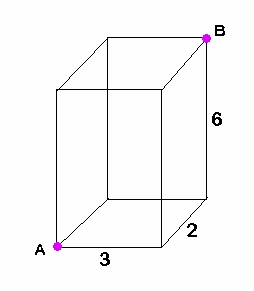# ACT, Geometry – diagonal of a rectangular prism

Q: What is the (straight-line) distance between points A and B on the figure below?Explanation:  We are finding the length of the green segment below:This requires the three-dimensional version of the distance formula:

There is another way to do this, using a progression of two right triangles, with the green segment being the hypotenuse of the second triangle, but I find the above formula much simpler.  It is just an extension of the ‘usual’ (2-dimensional) distance formula we are so familiar with from Algebra and Geometry.

BUT if you want an even easier way, and I know you do, use my TI-84 Plus program ‘DIST3D’.Lorem ipsum dolor sit amet, consectetur adipisicing elit, sed do eiusmod tempor incididunt ut labore et dolore magna aliqua. Ut enim ad minim veniam, quis nostrud exercitation ullamco laboris nisi ut aliquip ex ea commodo consequat.

# Trig / Precalculus – convert degrees to radians

Q: Convert from degrees to radians:  330°Explanation:My TI-84 Plus program ‘DEG2RAD’ will convert this for you.